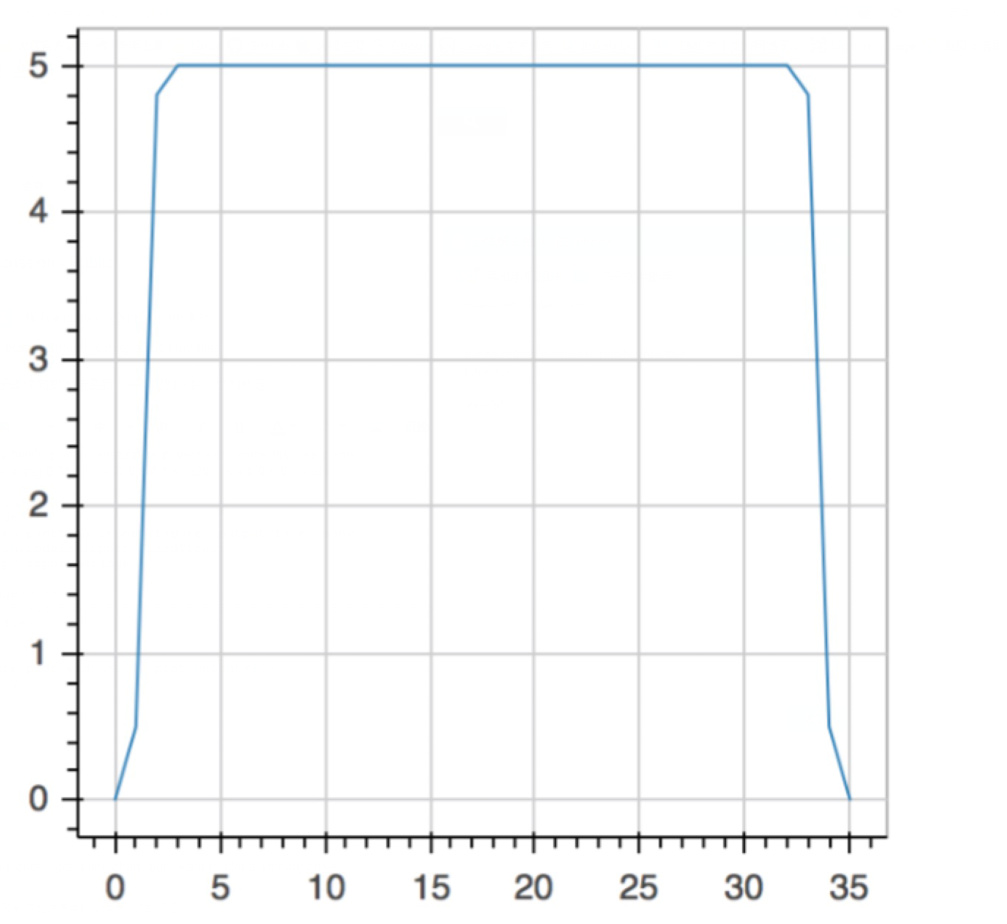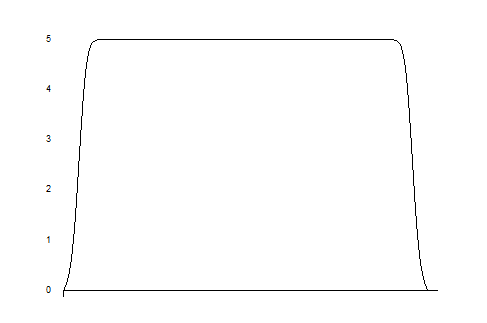# smooth cruve, static html

#!/usr/bin/python
#coding:utf-8
from bokeh.plotting import figure, output_file, show
from bokeh.models import FixedTicker
from numpy import arange

x = arange(0,36,1)
y = [0,0.5,4.8,5,5,5,5,5,5,5,5,5,5,5,5,5,5,5,5,5,5,5,5,5,5,5,5,5,5,5,5,5,5,4.8,0.5,0]
output_file(“fangbo.html”)

p = figure(plot_width=400, plot_height=400)
p.line(x,y)

show§

``

Hi,

I’m still new in bokeh.
I’m currently building on a visualization project with some discrete points,
such as x = arang(0,36,1) y = [0,0.5,4.8,5…(28)…5,4.8,0.5,0]，I use
Then run this code I got a line chart, but I want to plot a smooth cruveHow can I fit the cruve to make it smooth. I didn’t find answer in bokeh doc, so I hope solve this problem in here.

Thanks a lotShuai Yang

The only thing you can do to make it more smooth is to increase the number of points. You are currently using 36 points, but for the smoothness that you want, you’ll need 1 point per pixel. So, if your chart is 500 pixels wide, you’ll need x = linspace(0, 35, 500). (Of course, this assumes that the numerical domain of your function is [0,35].)

I’m not sure how you generated your Y values, but you’d need to regenerate them, or define a Python function which can actively and dynamically generate the values as you zoom.

-Peter···

On Fri, Jun 17, 2016 at 4:44 AM, Shuai Yang [email protected] wrote:

#!/usr/bin/python
#coding:utf-8
from bokeh.plotting import figure, output_file, show
from bokeh.models import FixedTicker
from numpy import arange

x = arange(0,36,1)
y = [0,0.5,4.8,5,5,5,5,5,5,5,5,5,5,5,5,5,5,5,5,5,5,5,5,5,5,5,5,5,5,5,5,5,5,4.8,0.5,0]
output_file(“fangbo.html”)

p = figure(plot_width=400, plot_height=400)
p.line(x,y)

show§

``

Hi,

I’m still new in bokeh.
I’m currently building on a visualization project with some discrete points,
such as x = arang(0,36,1) y = [0,0.5,4.8,5…(28)…5,4.8,0.5,0]，I use
Then run this code I got a line chart, but I want to plot a smooth cruve
How can I fit the cruve to make it smooth. I didn’t find answer in bokeh doc, so I hope solve this problem in here.

Thanks a lotShuai Yang

You received this message because you are subscribed to the Google Groups “Bokeh Discussion - Public” group.

To unsubscribe from this group and stop receiving emails from it, send an email to [email protected].

To post to this group, send email to [email protected].

To view this discussion on the web visit https://groups.google.com/a/continuum.io/d/msgid/bokeh/58b4d759-309b-4e9c-9b03-d09484d65168%40continuum.io.

Peter Wang

CTO, Co-founderThanks a lot for your answer. Now I have already known bokeh don’t have a function like “spcrv” in matlab, I will try to learn about interpolation with Python.

Finally, I am very sorry for not timely feedback, because the Great Firewall in China.

Shuai Yang

···

The only thing you can do to make it more smooth is to increase the number of points. You are currently using 36 points, but for the smoothness that you want, you’ll need 1 point per pixel. So, if your chart is 500 pixels wide, you’ll need x = linspace(0, 35, 500). (Of course, this assumes that the numerical domain of your function is [0,35].)

I’m not sure how you generated your Y values, but you’d need to regenerate them, or define a Python function which can actively and dynamically generate the values as you zoom.

-Peter

On Fri, Jun 17, 2016 at 4:44 AM, Shuai Yang [email protected] wrote:

#!/usr/bin/python
#coding:utf-8
from bokeh.plotting import figure, output_file, show
from bokeh.models import FixedTicker
from numpy import arange

x = arange(0,36,1)
y = [0,0.5,4.8,5,5,5,5,5,5,5,5,5,5,5,5,5,5,5,5,5,5,5,5,5,5,5,5,5,5,5,5,5,5,4.8,0.5,0]
output_file(“fangbo.html”)

p = figure(plot_width=400, plot_height=400)
p.line(x,y)

show§

``

Hi,

I’m still new in bokeh.
I’m currently building on a visualization project with some discrete points,
such as x = arang(0,36,1) y = [0,0.5,4.8,5…(28)…5,4.8,0.5,0]，I use
Then run this code I got a line chart

, but I want to plot a smooth cruveHow can I fit the cruve to make it smooth. I didn’t find answer in bokeh doc, so I hope solve this problem in here.

Thanks a lotShuai Yang

You received this message because you are subscribed to the Google Groups “Bokeh Discussion - Public” group.

To unsubscribe from this group and stop receiving emails from it, send an email to [email protected].

To post to this group, send email to [email protected].

To view this discussion on the web visit https://groups.google.com/a/continuum.io/d/msgid/bokeh/58b4d759-309b-4e9c-9b03-d09484d65168%40continuum.io.